# Sequences Worksheet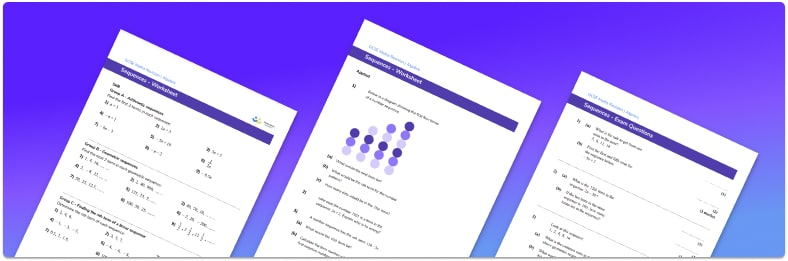Help your students prepare for their Maths GCSE with this free sequences worksheet of 30+ questions and answers

• Section 1 of the sequences worksheet contains 20+ skills-based sequences questions, in 3 groups to support differentiation
• Section 2 of the resource contains 3 applied sequences questions with a mix of worded problems and deeper problem solving questions
• Section 3 contains 3 foundation and higher level GCSE practice and exam questions
• Answers and a mark scheme for all sequences questions are provided
• Questions follow variation theory with plenty of opportunities for students to work independently at their own level
• All questions created by fully qualified expert secondary maths teachers
• Suitable for GCSE maths revision for AQA, OCR and Edexcel exam boards
• To receive this resource and regular emails with more free resources, blog posts and other Third Space updates, enter your email address and click below.

• This field is for validation purposes and should be left unchanged.

You can unsubscribe at any time (each email we send will contain an easy way to unsubscribe). To find out more about how we use your data, see our privacy policy.

### Sequences at a glance

Sequences are a set of numbers that have a starting number and go on forever. Number sequences can increase or decrease and can be whole numbers, fractions or decimals.

There are different ways to classify a sequence including arithmetic sequences and geometric sequences. Arithmetic sequences have a common difference and geometric sequences have a common ratio. The common difference or common ratio can be used to continue a sequence, or to find missing terms within the sequence and may be referred to as the term-to-term rule.

Arithmetic sequences are based on multiples which can be used to find the nth term rule (or nth term formula) by calculating the common difference by subtracting two consecutive terms in the sequence. The common difference gives the coefficient of the n in the nth term formula.

The nth term can be referred to as the position-to-term rule and can be used to find a particular term in the sequence or used to check if a particular value is in the sequence or not. A sequence that is increasing or decreasing by the same amount is called a linear sequence.

There are other types of sequences such as the square numbers that form a sequence which is involved in quadratic sequences. Other non-linear sequences include the cube numbers and the powers of 10. There is also the Fibonacci sequence where the next term is found by adding the previous two terms. Triangular numbers is another special sequence which is useful to know.

Looking forward, students can then progress to additional algebra worksheets, for example a straight line graph or simultaneous equations worksheet.For more teaching and learning support on Algebra our GCSE maths lessons provide step by step support for all GCSE maths concepts.

## Related worksheets

Quadratic Sequences and Quadratic Nth Term Worksheet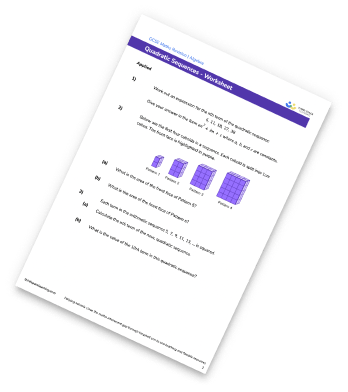Arithmetic Sequence Worksheet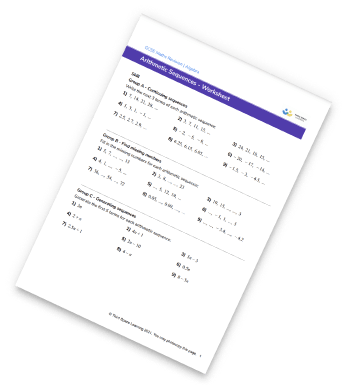Nth Term Worksheet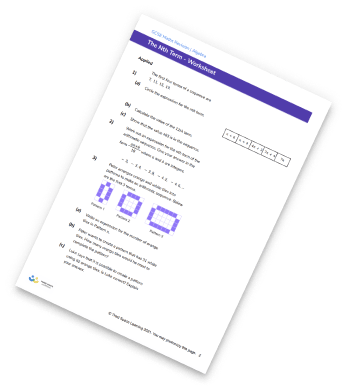Geometric Sequence Worksheet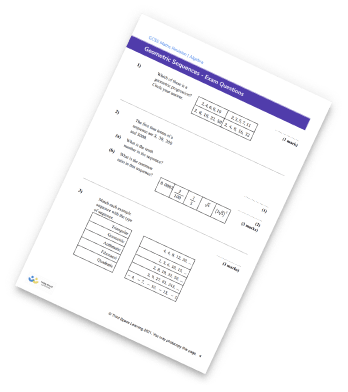## Do you have KS4 students who need more focused attention to succeed at GCSE?There will be students in your class who require individual attention to help them succeed in their maths GCSEs. In a class of 30, it’s not always easy to provide.

Help your students feel confident with exam-style questions and the strategies they’ll need to answer them correctly with our dedicated GCSE maths revision programme.

Lessons are selected to provide support where each student needs it most, and specially-trained GCSE maths tutors adapt the pitch and pace of each lesson. This ensures a personalised revision programme that raises grades and boosts confidence.

Find out more